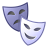# New to Qlik Sense

If you’re new to Qlik Sense, start with this Discussion Board and get up-to-speed quickly.

Announcements
cancel
Showing results for
Did you mean:Creator

## creating pie chart choosing multiple column

Hi,

wondering do you have any suggestion creating a pie chart using multiple column without change the data structure

Having data structure like this, need to create other dashboard so can not change the structure :Each row are each individual haveing A--G costs. and Total =sum(A:G) --Total is total cost

is there any chance can create pie chart for each individual t1, t2.....(will use filter for this so only need one pie chart)

showing the decomposition of the Total cost. So we do not need the total cost but have A:G in the same pie chart and see the %

here is the data (in the second sheet: name 'sheet 1')

Thank you.

1 Solution

Accepted SolutionsPartner

You can, but it would be nicer to change ur data structure..

What u can do without altering ur table is :

as dimension:

ValueList('B','C','D','E','F','G')

as a measure:

if(ValueList('B','C','D','E','F','G')='B', sum(B),

if(ValueList('B','C','D','E','F','G')='C', sum(C),

if(ValueList('B','C','D','E','F','G')='D', sum(D),

if(ValueList('B','C','D','E','F','G')='E', sum(E),

if(ValueList('B','C','D','E','F','G')='F', sum(F),

if(ValueList('B','C','D','E','F','G')='G', sum(G)

))))))

14 RepliesCreator II

Hi,

See if the attached works for you.

Thanks,

RTCreator
Author

H Rajesh,

Thank you. But this is not what I want.

what I want is

the Pie showing the proportion of B to G (with out A)

because B:G are columns instead of Row so I do not know how to create this pie.

you can have this pie for all the t. or only one single t

but showing the decomposition of how B,C,D,E,F,G to the sum(B,C,D,E,F,G)

Thank you!Partner

You can, but it would be nicer to change ur data structure..

What u can do without altering ur table is :

as dimension:

ValueList('B','C','D','E','F','G')

as a measure:

if(ValueList('B','C','D','E','F','G')='B', sum(B),

if(ValueList('B','C','D','E','F','G')='C', sum(C),

if(ValueList('B','C','D','E','F','G')='D', sum(D),

if(ValueList('B','C','D','E','F','G')='E', sum(E),

if(ValueList('B','C','D','E','F','G')='F', sum(F),

if(ValueList('B','C','D','E','F','G')='G', sum(G)

))))))Creator II

Hi,

Is this what you want?

Thanks,

RTCreator
Author

Yes. That is.Creator
Author

Hi Omar,

thank you could I create a 'new dimension' use the expression you suggestedand I will put in the Field area the function:

ValueList('B','C','D','E','F','G')

one question here is what the different using g ' ', and [ ]

I was using fx insert, then I got this

ValueList([B],, ,,,)

Thank you!Creator
Author

Seems not working

I have my real data title as

and I put the ValueList() dimention, it says not validCreator
Author

Hi Rajesh,

I copied your expression from the file to my real one and change the A,B,C,D to the name in my dataset.

have this

but I also get a the this --the red frame  (seems something wrong)any suggestion?

=Type

/*pick(match(Type,'New cars','Used cars','Service','Other Service','Spare parts','Bodyshop')

,New cars

,Used cars

,Service

,Other Service

,Spare parts

,Bodyshop

)

)*/Partner

can u click on the fx and let me see how it's written?